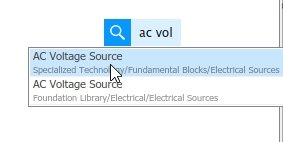# Solving RLC circuit using MATLAB Simulink : tutorial 5

In this tutorial, I will explain you the working of RC and RL circuit. First the brief and concise introduction of capacitive and inductive circuits is provided explaining the effect of introducing each of them in a resistive circuit. After that the concept provided in introduction part is implemented with the help of MATLAB’s Simulink, and the theoretical results are compared with the virtual results of the block diagram provided by Simulink which comes out to be same. At the end of the tutorial you are provided with an exercise to do it by yourself, and in the next tutorials I will assume that you have done those exercises and I will not explain the concept regarding them.

### How to solve RLC Circuits using Simulink

Lets’ now move towards the programming part. Up till now we have been using library browser to place any block in simulink for simulation purpose.  But that’s quite hectic to search for each block in the library browser in each section. We had another option to search for the block in the library browser, but that too was too much time consuming. Another and so far best option to place a block is to search for it in the simulink window. Just stay in the simulink window and press the search button from your keyboard. This will show a small search icon on the simulink window as shown in the figure below,Figure 1: Searching

• Click on that search sign and type the block name or a relevant name you want to search and a drop down menu will appear with a list of similar blocks showing, which are available in Simulink’s library browser. For instance, in our case we want to place an AC voltage source which will be used as an input source for the RLC circuit. Type ac voltage source in the search bar and a drop down with multiple items will appear as shown in the figure below,Figure 2: AC source search

Figure 3: AC voltage source

Figure 4: RLC branch search

Figure 5: Series RLC branch

Figure 6: 3 RLC branches

Figure 7: Resistor branch

Figure 8: Inductor branch

Figure 9: Capacitor branch

Figure 10: Changed branches

Figure 11: Voltage measurement search

Figure 12: Connected circuit

Figure 13: Ground search

Figure 14: Connected ground

Figure 15: Voltage measurement

Figure 16: Scope placement

Figure 17: Connected scopes

Figure 18: Configuration properties

Figure 19: Input ports

Figure 20: Multiple input scope

Figure 21: Powergui search

Figure 22: Powergui

Figure 23: Output

Figure 24: Legends

Figure 25: Layout

Figure 26: Layout blocks

Figure 27: Output graphs

Notice the amplitude of all the waveforms which are in accordance with the theory.

Exercise:

• Try to work with RL and RC circuit. Use the similar fashion as discussed here except using either inductor or capacitor in place of both.